# Chapter 5 Arithmetic Progressions NCERT Exemplar Solutions Exercise 5.1 Class 10 Maths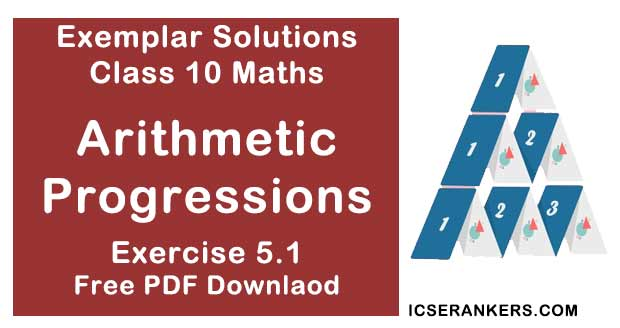Chapter Name NCERT Maths Exemplar Solutions for Chapter 5 Arithmetic Progressions Exercise 5.1 Book Name NCERT Exemplar for Class 10 Maths Other Exercises Exercise 5.1Exercise 5.2Exercise 5.3 Related Study NCERT Solutions for Class 10 Maths

### Exercise 5.1 Solutions

Multiple Choice Questions

Choose the correct answer from the given four options in the following questions:

1. In an AP, if d = -4, n = 7, an = 4, then a is
A. 6
B. 7
C. 20
D. 28

Solution

(D) 28
In an A.P,
an = a + (n - 1)d
(a = first term, an is nth term and d is the common difference)
4 = a + (7 - 1)(-4)
⇒ 4 = a - 24
⇒ a = 24 + 4 = 28

2. In an AP, if a = 3.5, d = 0, n = 101, then an will be
A. 0
B. 3.5
C. 103.5
D. 104.5

Solution

(B) 3.5
In an A.P,
an = a + (n - 1)d
(a = first term, an is nth term and d is the common difference )
an = 3.5 + (101 - 1)0 = 3.5
(Since, d = 0, it's a constant A.P)

3. The list of numbers -10, -6, -2, 2, ..... is
A. an AP with d = -16
B. an AP with d = 4
C. an AP with d = -4
D. not an AP

Solution

In the given A.P,
a1 = -10
a2 = -6
a3 = -2
a4 = 2
a2 - a1  = 4
a3 - a2 = 4
a4 - a3 = 4
a2 - a1 = a3 - a2 = a4 - a3  = 4
So, it is an A.P with d = 4

4. The 11th term of he A.P. :  -5, -5/2, 0, 5/2 , ....... is
A. -20
B.  20
C. -30
D. 30

Solution
According to the given A.P.
a = - 5
d = 5 - (-5/2) = 5/2
n = 11
Also,
an = a + (n - 1)d
Here, (a = first term, an  is nth term and d is the common difference)
a11 = -5+ (11 - 1)(5/2)
⇒ a11  = -5 + 25 = 20

5. The first four terms of an AP, whose first term is –2 and the common difference is –2, are
A. –2, 0, 2, 4
B. –2, 4, –8, 16
C. –2, –4, –6, –8
D. –2, –4, –8, –16

Solution

First term,
a = - 2
Second term,
d = -2
a1 = a = -2
Also,
an = a + (n - 1)d
where,
a = first term, an is nth term, d is the common difference
Therefore,
a2 = a + d
= -2 + (-2)  = - 4
Similarly,
a3  = -6
a4 = -8
So, the A.P is -2, -4, -6, -8.

6. The 21st term of the AP whose first two terms are -3 and 4 is
A. 17
B. 137
C. 143
D. -143

Solution

First two terms of an AP are a = -3 and a2 = 4.
We know, nth term of an AP is
an = a + (n - 1)d
Here, a = first term, an is nth term, d is the common difference
a2 = a + d
⇒ 4 = -3 + d
⇒ d = 7
Common difference,
d = 7
a21  = a + 20d
= -3 + 20 × 7
= 137

7. If the 2nd term of an AP is 13 and the 5th term is 25, what is its 7th term ?
A. 30
B. 33
C. 37
D. 38

Solution

In an A.P.
an = a + (n - 1)d
Here, a = first term, an is nth term, d is he common difference
a2 = a + d  = 13 ...(i)
a5 = a + 4d = 25 ...(ii)
From equation (i),
a = 13 - d
Using this in equation (ii),
13 – d + 4d = 25
⇒ 13 + 3d = 25
⇒ 3d = 12
⇒ d = 4
a = 13 – 4 = 9
a7  = a + 6d
= 9 + 6(4)
= 9 + 24 = 33

8. Which term of the AP. 21, 42, 63, 84.... is 210 ?
A. 9th
B. 10th
C. 11th
D 12th

Solution

Let nth term of the given AP be 210.
According to question,
First term,
a = 21
Common difference,
d = 42 – 21 = 21
an  = 210
We know that the nth term of an AP is an = a + (n – 1)d
Where, a = first term, an is nth term, d is the common difference
210 = 21 + (n – 1)21
⇒ 189 = (n – 1)21
⇒ n – 1 = 9
⇒ n = 10
So, 10th term of an AP is 210.

9. If the common difference of an AP is 5, then what is a18 - a13 ?
A. 5
B. 20
C. 25
D. 30

Solution
Given, d = 5
Now,
As we know, nth term of an AP is
an  = a + (n – 1)d
Here, a = first term, an is nth term, d is the common difference
a18 -a13 = a + 17d – (a + 12d)
= 5d
= 5(5)
= 25

10.  What is the common difference of an AP in which a18 - a14 = 32 ?
A. 8
B. -8
C. -4
D. 4

Solution
(a) a18 - a14 = 32
[a + (18 – 1)d] – [a + (14 – 1)d] = 32  [an = a + (n – 1)d]
a + 17d – a – 13d = 32
⇒ 4d = 32
⇒ d = 8
So, (a) is the correct answer.

11. Two APs have the same common difference. The first term of one of these is –1 and that of the other is – 8. Then the difference between their 4th terms is
A. -1
B. -8
C. 7
D. -9

Solution

(C)
According to question,
a1 (for the first AP) = -1 and a1 (for the second AP) = -8
Let d be the same common difference of two A.Ps.
d1 = d,
an = a + (n - 1)d
Now,
a4 of first AP - a4 of second AP
(-1 + 3d) - (-8 + 3d)
⇒ -1 + 3d + 8- 3d = 7
So, the required answer is (c).

12. If 7 times the 7th term of an AP is equal to 11 times its 11 th term, then its 18th term will be
A. 7
B. 11
C. 18
D. 0

Solution

(d)
a18 = a + (18 – 1)d
= a + 17d
Also,
7a7  = 11a11  (Given)
⇒ 7[a + (7 – 1)d] = 11[a + (11 – 1)d]
⇒ 7[a + 6d] = 11[a + 10d]
⇒ 7a + 42d = 11a + 110d
⇒ 0 = 11a – 7a + 110d – 42d
⇒ 0 = 4a + 68d
⇒ 0 = a + 17d
⇒ a18  = 0
So, (d) is the correct answer.

13. The 4th term from the end of the AP: –11, –8, –5, ..., 49 is
A. 37
B. 40
C. 43
D. 58

Solution

Reversing the A.P., we get
49 ......, -5, -8, and -11
d = -8 - (-5)
= -8 + 5 = -3
a = 49 and n = 4
an = a + (n - 1)d
⇒ a4 = 49 + (4 - 1)(-3)
⇒ a4 = 49 + 3(-3)
= 49 - 9
⇒ a4 = 40
So, the required value of a4 is 40 and answer is (b)

14. The famous mathematician associated with finding the sum of the first 100 natural numbers is
A. Pythagoras
B. Newton
C. Gauss
D. Euclid

Solution
(C)
Gauss is the famous mathematician associated with finding the sum of first 100 natural numbers, i.e., 1 + 2 + 3 + 4 + 5 + ... + 100
a = 1, d = 1, n = 100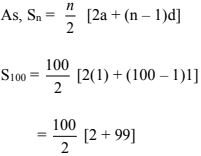= 50 × 101
= 5050

15. If the first term of an AP is –5 and the common difference is 2, then the sum ofthe first 6 terms is
A. 0
B. 5
C. 6
D. 15

Solution

(a)
a = -5,
d = 2
n = 6
We have,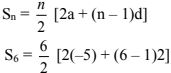= 3[-10 + 5 × 2]
= 3[ -10 + 10]
= 3
S6 = 0
So, (a) is the correct answer.

16. The sum of first 16 terms of the AP: 10, 6, 2,... is
A. –320
B. 320
C. –352
D. –400

Solution

(a)
a = 10,
n = 16,
d = 6 – 10 = –4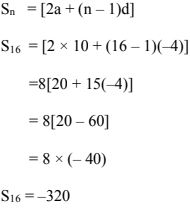So, the required answer is (a)

17. If AP if a = 1 an = 20 and Sn = 399, then n is
A. 19
B. 21
C. 38.
D. 42

Solution
(c)
Sn = [2a + (n - 1)d]
Sn = [a + a + (n - 1)d]
⇒ 399 = [a + an ]   [an = last term]
⇒ 399 = [1 + 20]
⇒ n = 38
So, (c) is the correct answer.

18. The sum of first five multiples of 3 is
A. 45
B. 55
C. 65
D. 75

Solution

(a)
1st five multiples of 3 are 3, 6, 9, 12, 15, .....
a = 3,
n = 5,
d = 6 – 3 = 3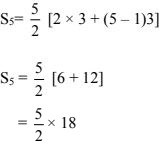= 45